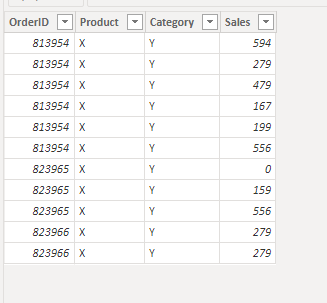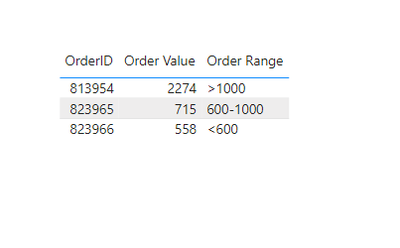cancel
Showing results for
Did you mean:Helper I

## Create order value buckets from dataset with order rows

Hi!

I have a dataset with order rows, with a unique ID for each order. I want to be able to create a column/tag for each orderID with a designated order value range.

See below for how the dataset looks like and what I would like to achieve.Any ideas?

1 ACCEPTED SOLUTIONCommunity Support

Hi @ErikOmni ,

1. below is my test table

Table:2. create a measure with below dax formula

``````Order Range =
VAR cur_sale =
CALCULATE (
SUM ( 'Table'[Sales] ),
'Table'[OrderID] = SELECTEDVALUE ( 'Table'[OrderID] )
)
RETURN
SWITCH (
TRUE (),
cur_sale > 1000, ">1000",
cur_sale > 600
&& cur_sale < 1000, "600-1000",
cur_sale < 600, "<600"
)
``````

3. add a table visual with fields and measurePlease refer the attached .pbix file.

Best regards,
Community Support Team_Binbin Yu
If this post helps, then please consider Accept it as the solution to help the other members find it more quickly.

3 REPLIES 3Community Support

Hi @ErikOmni ,

1. below is my test table

Table:2. create a measure with below dax formula

``````Order Range =
VAR cur_sale =
CALCULATE (
SUM ( 'Table'[Sales] ),
'Table'[OrderID] = SELECTEDVALUE ( 'Table'[OrderID] )
)
RETURN
SWITCH (
TRUE (),
cur_sale > 1000, ">1000",
cur_sale > 600
&& cur_sale < 1000, "600-1000",
cur_sale < 600, "<600"
)
``````

3. add a table visual with fields and measurePlease refer the attached .pbix file.

Best regards,
Community Support Team_Binbin Yu
If this post helps, then please consider Accept it as the solution to help the other members find it more quickly.Resident Rockstar

Create measures like below,

• Order Value
``Order Value = SUM(tablename[Sales])``
• Order Range
``````Order Range = SWITCH(
TRUE(),
[Order Value] > 1000, "> 1000",
AND([Order Value] > 600, [Order Value] < 1000),"600-1000",
"< 600"
)``````Helper I

@v-binbinyu-msft Hi! Sorry for late reply. The solution worked, however, I realized I need it to be column to allow me to track YoY development into different buckets.

This formula give me a circular reference warning when I try to apply it as a measure.